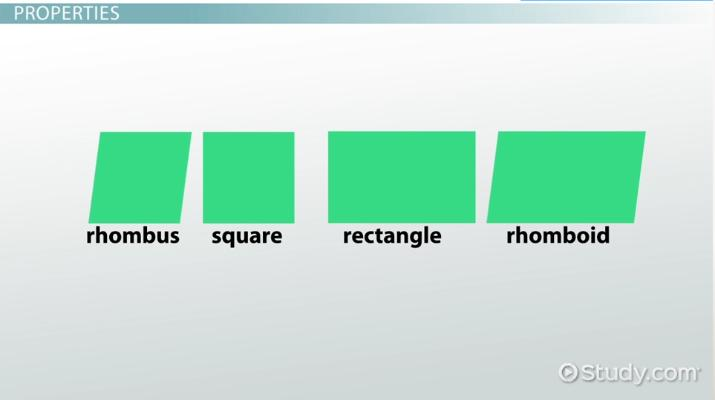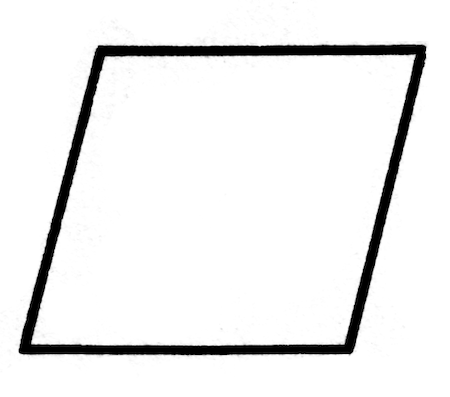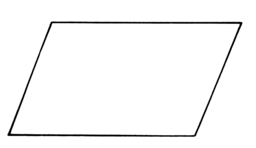# Parallelogram Definition and Examples

Louise Fisher, Cathryn Jackson, Kathryn Boddie
• Author
Louise Fisher

Louise Fisher has taught middle school students introductory physics topics for two years. She earned her bachelor's in Physics and Astronomy from the University of North Carolina at Asheville. She currently works as a physicist assistant at a cancer treatment center.

• Instructor
Cathryn Jackson

Cat has taught a variety of subjects, including communications, mathematics, and technology. Cat has a master's degree in education and is currently working on her Ph.D.

• Expert Contributor
Kathryn Boddie

Kathryn has taught high school or university mathematics for over 10 years. She has a Ph.D. in Applied Mathematics from the University of Wisconsin-Milwaukee, an M.S. in Mathematics from Florida State University, and a B.S. in Mathematics from the University of Wisconsin-Madison.

What is a parallelogram? Learn the definition of the parallelogram shape in geometry. Read about the properties of parallelograms and see examples. Updated: 08/27/2021

Show

## What Is a Parallelogram?

Parallelograms are found everywhere in nature and in geometry. They are a specific classification for shapes with four sides. Notebooks, cell phones, and keyboards are all real-world examples of shapes that lie within the parallelogram classification.

The definition of a parallelogram is a geometric shape with four sides, and, as the name suggests, two sets of parallel lines. All parallelograms have exactly four corners, four angles, and four sides. Since they have four sides, it is considered a type of quadrilateral. Quadrilaterals are shapes with four total sides. Parallelograms will have either the same length for every side or two different side lengths (one length for each pair of parallel lines). Parallelograms will never have more than two length values for the sides, or they wouldn't be considered a parallelogram. If three of the four sides had different lengths, then one of the line pairs wouldn't be parallel. Therefore, it is not possible for there to be more than two side lengths if there are two sets of parallel lines.Notice that the illustration has two sets of parallel sides and two side length values. It also has exactly four corners, angles, and sides. It wouldn't be possible for a shape to contain four sides with two sets of parallel lines and three different lengths.An error occurred trying to load this video.

Try refreshing the page, or contact customer support.

Coming up next: Planes and the Polyhedron: Definition and Example

### You're on a roll. Keep up the good work!

Replay
Your next lesson will play in 10 seconds
• 0:01 What Is a Parallelogram?
• 1:02 Properties
• 1:39 Lesson Summary
Save Save

Want to watch this again later?

Timeline
Autoplay
Autoplay
Speed Speed

## Types of Parallelogram Shapes

There are a few different quadrilateral shapes that are considered to be parallelograms. These shapes include squares, rectangles, rhombuses, and rhomboids. All of these shapes have four sides, four corners, and four angles. They also all have two sets of parallel lines, making them parallelograms.

### Square

A square is a shape with four sides of equal length. They also have four 90° angles (or four right angles); One right angle located at each corner. Squares are categorized as being parallelograms because they are quadrilaterals with two sets of parallel lines.The shape in the image has four sides, making it a quadrilateral. Since it has four right angles and four sides of equal lengths, it is a square. It also has two sets of parallel lines opposite of one another, making it a parallelogram.

### Rectangle

Rectangles are a type of quadrilateral with two different side lengths. Since they are quadrilateral, they have four sides, four corners, and four angles. All of the angles in a rectangle are right angles, similar to squares. Unlike squares, however, not all of the side lengths are equal. Two of the sides will be a different length than the other two.Notice how not all the sides are the same length, but there are still four right angles at each corner. A rectangle is still considered a parallelogram because it has two sets of lines parallel opposite one another. The sides opposite of another also have the same length values.

### Rhombus

A rhombus is a type of shape with four sides, making it a quadrilateral. Similar to a square, a rhombus is classified by having four sides of the same length. The difference, however, is that a rhombus doesn't have four right angles or any right angles at all. Rhombuses instead have two acute and two obtuse angles, each directly across from each other. This makes the shape appear like a slanted square since the sides are slanted at an angle. A diamond is an example of a rhombus, as it has four sides of equal length, but they are slanted. Since a diamond is a rhombus, and a rhombus is a parallelogram, then a diamond is also a parallelogram.The illustration displays a shape with four sides of the same length, but no right angles at the corners. This makes the shape a rhombus since there are four sides of equal length but slanted. Notice how the acute and obtuse angles are across from one another. There are also two separate sets of parallel lines, making this shape a parallelogram.

### Rhomboid

The last type of parallelogram is called a rhomboid. As the name hints, a rhomboid is very similar to a rhombus. It's also very similar to a rectangle. Rhomboids have four sides, making them quadrilateral. However, unlike a rhombus, a rhomboid doesn't have four sides of the same length. Two of the sides have a different length than the other two (similar to a rectangle). They don't have any right angles though, making them different than rectangles. They have two acute and two obtuse angles opposite of each other.A rhomboid can be thought of like a rectangle with slanted sides. Since they consist of two pairs of parallel lines, they are classified as parallelograms. Notice how this rhomboid has two acute and two obtuse angles opposite from one another. This is one of the characteristics of rhomboids.This chart illustrates how each of these shapes relates to the others. All of the shapes have four sides, four corners, and four angles. This makes all of the shapes quadrilaterals. A rhombus and a square are similar because they both have four sides of equal length. A rectangle and a rhomboid are similar because they have two different side lengths. All of the shapes are parallelograms because they all have two sets of parallel lines and four total sides.

## Properties of Parallelograms

There are specific properties of parallelograms that make them geometrically unique. When followed, these guidelines can determine whether or not a shape is considered a parallelogram.

To unlock this lesson you must be a Study.com Member.

• Activities
• FAQs

## Observing Parallelogram Properties - Activity

In the following activity, students will verify the properties of parallelograms through measurements. The hands-on measuring will help learners remember the properties of parallelograms.

## Materials

• Various parallelograms on paper - include rectangles, squares, rhombuses, and rhomboids.
• Ruler
• Protractor
• Pencil or pen

## Instructions

1. For each parallelogram, measure the opposite sides with the ruler. What do you observe?

2. For each parallelogram, measure the opposite angles with the protractor (opposite angles diagonally - that is if the upper left angle is measured, the lower right angle should be measured.) What do you observe?

3. For each parallelogram, measure the angles which share a side and write down the measurements (there should be 4 pairs of angles to write down). What do you observe?

4. Use the ruler to draw the two diagonals of the parallelograms. Then, measure the length of each diagonal from a corner to the point of intersection in the middle. What do you observe?

## Guide to Activity

Students should observe that the opposite sides of the parallelograms are congruent - even if all four sides are not. Similarly, they should observe that the opposite angles in a parallelogram are congruent - even if all four angles are not congruent. When measuring angles which share a side, if students do not notice any relationship, suggest they try different operations on the angles - the goal is to note that the sum of the angles which share a side is always equal to 180 degrees. When measuring the pieces of the diagonals, students should observe that the diagonals are divided perfectly in half.

#### What are the properties of a parallelogram?

Parallelograms must have two sets of parallel lines, with four lines total. At least one pair of the lines will have equal length. Lines drawn diagonally from the corners will create bisecting lines.

#### Is parallelogram a rectangle?

A rectangle is considered a parallelogram. It has two pairs of parallel lines, and at least one of the parallel lines is the same length, making it a parallelogram.

#### Do parallelograms have 4 equal sides?

Although they can, not all parallelograms have four equal sides. They will, however, have at least two sides of the same length.

#### What are the 4 types of parallelograms?

There are four types of parallelograms and include squares, rectangles, rhombuses, and rhomboids. These shapes are considered to be parallelograms because they have two sets of parallel lines.

#### Why is a square a parallelogram?

A square is considered a parallelogram because it consists of two sets of parallel lines, and at least one pair of parallel lines are the same length.

### Register to view this lesson

Are you a student or a teacher?